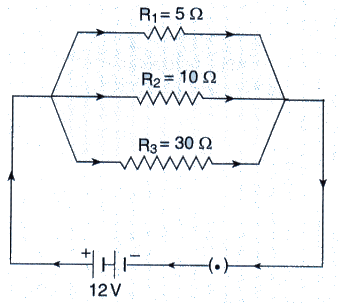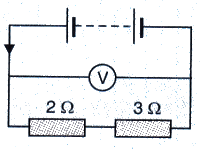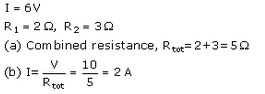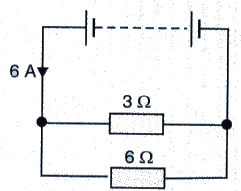# Solutions of Electricity (Page No- 40) - Physics By Lakhmir Singh, Class 10 Class 10 Notes | EduRev

## Class 10 : Solutions of Electricity (Page No- 40) - Physics By Lakhmir Singh, Class 10 Class 10 Notes | EduRev

The document Solutions of Electricity (Page No- 40) - Physics By Lakhmir Singh, Class 10 Class 10 Notes | EduRev is a part of the Class 10 Course Class 10 Physics Solutions By Lakhmir Singh & Manjit Kaur.
All you need of Class 10 at this link: Class 10

Lakhmir Singh Physics Class 10 Solutions Page No:40

Question 24:
In the circuit diagram given below, three resistors R1, R2, and R3 of 5 Ω, 10 Ω and 30 Ω, respectively are connected as shown.Calculate :
(a) current through each resistor.
(b) total current in the circuit.
(c) total resistance in the circuit.

Solution :

V = 12V
R1, R2 and R3 are connected in parallel.
(a) Current through R1 = V/R1 = 12/5 = 2.4 A

Current through R2 = V/R2 = 12/10 = 1.2 A

Current through R3 = V/R2 = 12/30 = 0.4A

(b) Total current in the circuit = 2.4 + 1.2 + 0.4 = 4 A

(c) Total resistance in the circuit=R

1/R = 1/R1+ 1/R2+ 1/R3
1/R = 1/5 + 1/10 + 1/30
1/R = 10/30
R = 3 ohm

Question 25:
A p.d. of 4 V is applied to two resistors of 6 Ω and 2 Ω connected in series. Calculate :
(a) the combined resistance
(b) the current flowing
(c) the p.d. across the 6 Ω resistor

Solution :

V = 4V.

R= 6 ohm. R2 = 8 ohm (in series)

(a) Combined resistance. R = R1 + R2 = 6+2 = 8ohm

(b) Current flowing. I = V/R = 4/8=0.5amp

(c) p.d. across 6ohm resistor = I x R1 = 0.5 x 6 = 3 V

Question 26:
A p.d. of 6 V is applied to two resistors of 3 Ω and 6 Ω connected in parallel. Calculate :
(a) the combined resistance
(b) the current flowing in the main circuit
(c) the current flowing in the 3 Ω resistor.

Solution :

V = 6V

R1= 3 ohm. R2 = 6 ohm (in parallel)

(a) Combined resistance.

1/R = 1/R1+ 1/R2

1/R = 1/3 + 1/6 = 3/6 = 1/2

R = 2 ohm

(b) Current flowing in the main circuit. I = V/R = 6/2 = 3 A

(c) Current flowing in 3 ohm resistor = V/R1= 6/3 = 2 A

Question 27:
In the circuit shown below, the voltmeter reads 10 V.(a) What is the combined resistance ?
(b) What current flows ?
(c) What is the p.d. across 2 Ω resistor ?
(d) What is the p.d. across 3 Ω resistor ?

Solution :(c) p.d. across 2Ω resistor=1 xR= 2 x 2 = 4V
(d) p.d. across 3Ω resistor=1 x R2 = 2 x 3 = 6V

Question 28:
In the circuit given below :(a) What is the combined resistance ?
(b) What is the p.d. across the combined resistance ?
(c) What is the p.d. across the 3 Ω resistor ?
(d) What is the current in the 3 Ω resistor ?
(e) What is the current in the 6 Ω resistor ?

Solution :

Total current flowing through circuit. I = 6 A

R1 = 3 ohm. R2 = 6 ohm

(a) Combined resistance R is

1/R = 1/3+1/6

1/R = 3/6

R = 2ohms

(b) p.d. across the combined resistance = IR = 6 x 2 = 12 V

(c) p.d. across the 3 ohm resistor = p.d. across the combined resistance = 12 V

(d) Current flowing through the 3 ohm resistor = V/R1 = 12/3 = 4 A

(e) Current flowing through the 6 ohm resistor = V/R2 = 12/6 = 2 A

Offer running on EduRev: Apply code STAYHOME200 to get INR 200 off on our premium plan EduRev Infinity!

94 docs

,

,

,

,

,

,

,

,

,

,

,

,

,

,

,

,

,

,

,

,

,

,

,

,

;